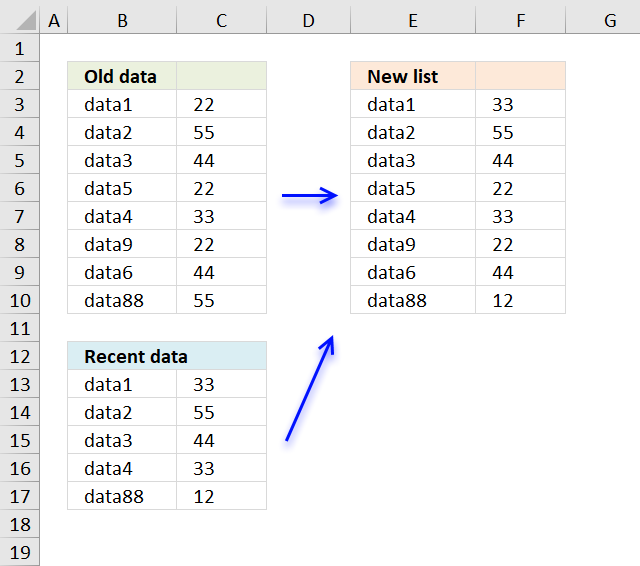Author: Oscar Cronquist Article last updated on December 05, 2018Question: i have two sets of data - one has an identifier column and one result column.

A2 data1 B2 22
A3 data2 B3 55
A4 data3 B4 44
A5  data5 B5 22
A6 data4 B6 33
A7 data9 B7 22
A8 data6 B8 44
A9 data88 B9 55

in the second set

D2 data1 E2 33
D3 data2 E3 55
D4 data3 E4 44
D5 data4 E5 33
D6 data88 E6 12

the new list should change B2 from 22 to 33.
If there is no change it shows the first sets result . if there is a change it reports from the second set.
To complicate it... the first set of data is twice as long as the second one. not all data is in the second set and finally the lists are not sorted and cant be.

Formula in F3:

=IF(COUNTIF(\$B\$13:\$B\$17, E3)=0, INDEX(\$C\$3:\$C\$10, MATCH(E3, \$B\$3:\$B\$10, 0)), INDEX(\$C\$13:\$C\$17, MATCH(E3, \$B\$13:\$B\$17, 0)))

copied down as far as needed.

### Explaining formula in cell B13

#### Step 1 - Count given value in cell range

The COUNTIF function counts cells based on a condition, it will return 1 if the value exists in cell range \$B\$13:\$B\$17.

COUNTIF(\$B\$13:\$B\$17, E3)=0

becomes

COUNTIF({"data1";"data2";"data3";"data4";"data88"},"data1")=0

becomes

1=0 and returns FALSE.

#### Step 2 - Which cell range?

The IF function determines from which cell range the formula retrieves the value needed.

IF(COUNTIF(\$B\$13:\$B\$17, E3)=0, INDEX(\$C\$3:\$C\$10, MATCH(E3, \$B\$3:\$B\$10, 0)), INDEX(\$C\$13:\$C\$17, MATCH(E3, \$B\$13:\$B\$17, 0)))

becomes

IF(FALSE, INDEX(\$C\$3:\$C\$10, MATCH(E3, \$B\$3:\$B\$10, 0)), INDEX(\$C\$13:\$C\$17, MATCH(E3, \$B\$13:\$B\$17, 0)))

and returns

INDEX(\$C\$13:\$C\$17, MATCH(E3, \$B\$13:\$B\$17, 0))

#### Step 3 - Get value

The MATCH function returns the relative position of a value in a cell range or array.

INDEX(\$C\$13:\$C\$17, MATCH(E3, \$B\$13:\$B\$17, 0))

becomes

INDEX(\$C\$13:\$C\$17, 1)

The INDEX function returns a value based on a row number (and a column number if needed).

INDEX(\$C\$13:\$C\$17, 1)

returns 33 in cell F3.